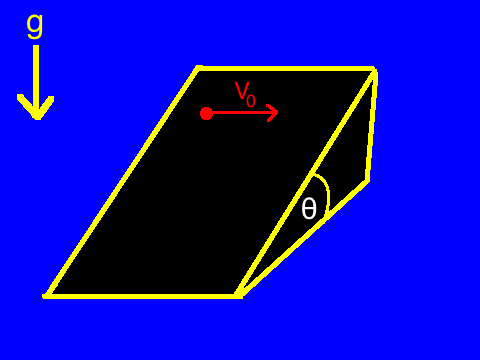# Terminal velocity of an object on an inclined plane?An object is on an inclined plane of angle $\theta$ with the horizontal. It is given an initial velocity of $V_0$ in the sideways direction (i.e. perpendicular to the down-hill direction). If the coefficient of friction is $\mu=\tan\theta$, then find the terminal velocity of the object. (Neglect air resistance.)

If the terminal velocity can be expressed as $V_{\text{terminal}}=kV_0$ and $k\geqslant 0$, then find $k$.

×